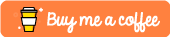# Foreground Segmentation using Python, OpenCV and Mediapipe

## Introduction

Hello! In this tutorial I will show you how to insert yourself into the background of another image. To do this we will need to use MediaPipes selfie segmentation.

## Installing the requirements

First we need to install the requirements needed for this project, create a file called "requirements.txt" and fill it with the following contents:

``````mediapipe
numpy
opencv-python
``````

Next, run the following command to install the dependencies:

``````pip3 install -r requirements.txt
``````

## Writing the code

Next we will write the code for the project, create a file called "main.py", open it and import the requirements needed:

``````import argparse
import cv2
import numpy as np
import mediapipe as mp
``````

Next we will create the main function:

``````if __name__ == "__main__":
ap = argparse.ArgumentParser()
ap.add_argument("-p", "--person", required = True, help = "path to image file of person")
ap.add_argument("-b", "--background", required = True, help = "path to background image")
args = vars(ap.parse_args()
``````

Here we create the main function and we also parse 2 arguments using argument parser, one being the image of the person, and the other being the background image we want the person to appear on.

Next we need to read the images passed to the program:

``````image = cv2.imread(args["person"])
``````

Next in order to get decent results we need to make sure the images are the same size which can be done via:

``````resized_bg_img = cv2.resize(background_img, (image.shape, image.shape))
``````

Now we can initialize mediapipe:

``````mp_selfie_segmentation = mp.solutions.selfie_segmentation
segment = mp_selfie_segmentation.SelfieSegmentation(model_selection = 0)
``````

We initialize Selfie Segmentation with a model_selection of 0, this model works best for faces that are within 2 meters. You can use 1 if your require up to 5 meters, but I've never heard of a 5 meter selfie.

Next we need to create the person's segmentation mask, we also need to convert the colors. This can be done via:

``````image = cv2.cvtColor(image, cv2.COLOR_BGR2RGB)

results = segment.process(image)

image = cv2.cvtColor(image, cv2.COLOR_RGB2BGR)

``````

This will cut the person out of the original image so it can be placed on the background image. Finally we can apply the segmentation mask to the background image via the following:

``````threshold = 0.5

``````

Please not that the results will vary depending on what the threshold is set to.

All we need to do now is save the results:

``````cv2.imwrite("result.jpg", replaced_img)
``````

Done, if you open up the image you should find that the person has appeared on the background image.

For the record these are the results I got: I also tried it on my cat.## Conclusion

Here I have shown how to perform foreground segmentation using a selfie and a background image.

Feel free to try it on numerous images, also depending on the sources you may need to change the threshold variable.

The repository for this tutorial can be found via: https://github.com/ethand91/opencv-segmentation

Like me work? I post about a variety of topics, if you would like to see more please like and follow me. Also I love coffee.